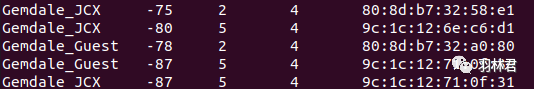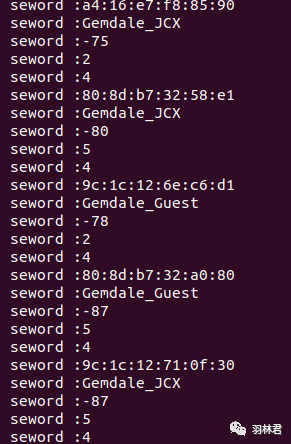+关注继续查看

# 前言：int i, j ;
i = 0;
j = strlen(strIn) - 1;
while(strIn[i] == ' ' || strIn[i] == '\t')
++i;

while(strIn[j] == ' ' || strIn[j] == '\t')
--j;
strncpy(strOut, strIn + i , j - i + 1);

# 解析代码

### 实际C++代码如下：

vector<string> Parse_Wifi_List(string& msg)
{
vector<string> words;
if(msg.empty())
return words;
string::iterator temp_p = msg.begin();
string sepword;
bool bit_true = false;
while(temp_p != msg.end())
{
if(/* * temp_p == ' ' || */  * temp_p == '\t' || * temp_p == '\n')
{
if(bit_true)
{
words.push_back(sepword);
PP_INFO("seword :%s",sepword.c_str());
sepword.clear();
}
bit_true = false;
temp_p++;
continue;
}
else
{
bit_true = true;
sepword += *temp_p;
}
if(*temp_p ++ == '\0')
{
break;
}
// else
// {
//  PP_INFO("%d %d %c",msg.end(),*temp_p,*temp_p);
// }
}
// for(auto point : words)
// {
//  printf(".%s\n",point.c_str());
// }
//for(uint16_t i =0;i<words.size();i+=5)
//{
// printf("[]%s,%s\n",words[i].c_str(),words[i+1].c_str(),words[i+3].c_str());
//}
return words;
}

### 使用此代码解析LOG如下：### 结语【查找算法】解析学习四大常用的计算机查找算法 | C++

22 0【一、构造函数与析构函数】深度解析C++类的构造函数与析构函数调用机制
【一、构造函数与析构函数】深度解析C++类的构造函数与析构函数调用机制
58 0TensorFlow Lite开发系列之C++接口解析(二)
TensorFlow Lite开发系列之C++接口解析
32 038 0c++json构建与解析组件 RapidJSON 没用过永远不会知道有多好用
c++json构建与解析组件 RapidJSON 没用过永远不会知道有多好用
64 0【力扣】1106. 解析布尔表达式（C++/Go 栈的应用）
【力扣】1106. 解析布尔表达式（C++/Go 栈的应用）
29 0C/C++ Qt 运用JSON解析库 [解析篇]
JSON是一种简单的轻量级数据交换格式，Qt库为JSON的相关操作提供了完整的类支持，使用JSON解析文件之前需要先通过TextStream流将文件读入到字符串变量内，然后再通过QJsonDocument等库对该JSON格式进行解析，以提取出我们所需字段。
122 0

C++ 入门教程开发文档1714042